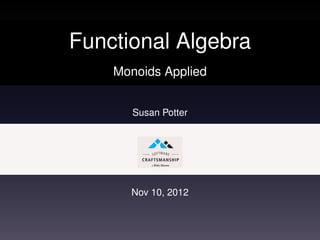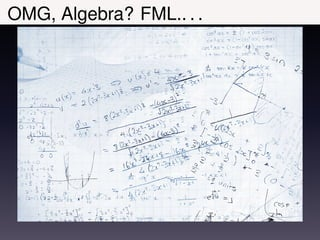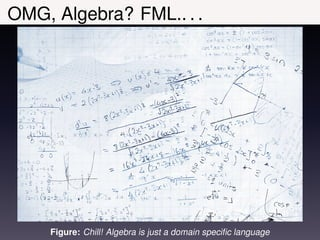Successfully reported this slideshow.×
1 of 47

Functional Algebra: Monoids Applied

13

Share

In functional programming, words from Category Theory are thrown around, but how useful are they really?
This session looks at applications of monoids specifically and how using their algebraic properties offers a solid foundation of reasoning in many types of business domains and reduces developer error as computational context complexity increases.
This will provide a tiny peak at Category Theory's practical uses in software development and modeling. Code examples will be in Haskell and Scala, but monoids could be constructed in almost any language by software craftsmen and women utilizing higher orders of reasoning to their code.

See all

See all

Functional Algebra: Monoids Applied

1. 1. Functional Algebra Monoids Applied Susan Potter Nov 10, 2012
2. 2. OMG, Algebra? FML.. . .
3. 3. OMG, Algebra? FML.. . . Figure: Chill! Algebra is just a domain speciﬁc language
4. 4. % whoami Figure: From OO patterns to FP abstractions
5. 5. Monoids: What are they? An abstraction (laws) not a design pattern (forces, context, . . . ) Algebraic structure . . . over a set with a binary operator and an identity element Accumulator . . . Its sole purpose Special case category . . . with only one object
6. 6. Monoids: What are they? An abstraction (laws) not a design pattern (forces, context, . . . ) Algebraic structure . . . over a set with a binary operator and an identity element Accumulator . . . Its sole purpose Special case category . . . with only one object
7. 7. Monoids: What are they? An abstraction (laws) not a design pattern (forces, context, . . . ) Algebraic structure . . . over a set with a binary operator and an identity element Accumulator . . . Its sole purpose Special case category . . . with only one object
8. 8. Monoids: What are they? An abstraction (laws) not a design pattern (forces, context, . . . ) Algebraic structure . . . over a set with a binary operator and an identity element Accumulator . . . Its sole purpose Special case category . . . with only one object
9. 9. Monoids: Typeclasses Listing 1: Haskell Monoid Typeclass Deﬁnition 1 -- | In Haskell Prelude Data. Monoid 2 class Monoid a where 3 mempty :: a -- identity 4 mappend :: a -> a -> a -- binary op 5 mconcat :: [a] -> a -- helper Listing 2: Scalaz Monoid Trait Deﬁnition 1 // Scalaz 7’s Monoid typeclass definition , kinda 2 trait Monoid [A] extends Semigroup [A] { self => 3 def zero: A /* identity */ 4 def append(x: A, y: => A): A /* binary op */ 5 } 6 // from SemigroupOps [A] ... 7 final def |+|(other: => A): A = A.append(self, other)
10. 10. Monoids: Typeclasses Listing 3: Haskell Monoid Typeclass Deﬁnition 1 -- | In Haskell Prelude Data. Monoid 2 class Monoid a where 3 mempty :: a -- identity 4 mappend :: a -> a -> a -- binary op 5 mconcat :: [a] -> a -- helper Listing 4: Scalaz Monoid Trait Deﬁnition 1 // Scalaz 7’s Monoid typeclass definition , kinda 2 trait Monoid [A] extends Semigroup [A] { self => 3 def zero: A /* identity */ 4 def append(x: A, y: => A): A /* binary op */ 5 } 6 // from SemigroupOps [A] ... 7 final def |+|(other: => A): A = A.append(self, other)
11. 11. Monoids: Typeclasses Listing 5: Haskell Monoid Typeclass Deﬁnition 1 -- | In Haskell Prelude Data. Monoid 2 class Monoid a where 3 mempty :: a -- identity 4 mappend :: a -> a -> a -- binary op 5 mconcat :: [a] -> a -- helper Listing 6: Scalaz Monoid Trait Deﬁnition 1 // Scalaz 7’s Monoid typeclass definition , kinda 2 trait Monoid [A] extends Semigroup [A] { self => 3 def zero: A /* identity */ 4 def append(x: A, y: => A): A /* binary op */ 5 } 6 // from SemigroupOps [A] ... 7 final def |+|(other: => A): A = A.append(self, other)
12. 12. Monoids: Typeclasses Listing 7: Haskell Monoid Typeclass Deﬁnition 1 -- | In Haskell Prelude Data. Monoid 2 class Monoid a where 3 mempty :: a -- identity 4 mappend :: a -> a -> a -- binary op 5 mconcat :: [a] -> a -- helper Listing 8: Scalaz Monoid Trait Deﬁnition 1 // Scalaz 7’s Monoid typeclass definition , kinda 2 trait Monoid [A] extends Semigroup [A] { self => 3 def zero: A /* identity */ 4 def append(x: A, y: => A): A /* binary op */ 5 } 6 // from SemigroupOps [A] ... 7 final def |+|(other: => A): A = A.append(self, other)
13. 13. Monoids: Laws Closure: ∀a, b ∈ S : ab ∈ S for all a and b in set S, the result of a and b given to the binary operator is also in set S. Associativity: ∀a, b, c ∈ S : (ab)c = a(bc) for all a, b, and c in set S, either binary operator can be evaluated ﬁrst to produce same result. Identity: ∃e ∈ S : ∀a ∈ S : ea = a = ae there exists an e in set S such that for all a in set S ea evaluates to a and is equal to ae
14. 14. Monoids: Laws Closure: ∀a, b ∈ S : ab ∈ S for all a and b in set S, the result of a and b given to the binary operator is also in set S. Associativity: ∀a, b, c ∈ S : (ab)c = a(bc) for all a, b, and c in set S, either binary operator can be evaluated ﬁrst to produce same result. Identity: ∃e ∈ S : ∀a ∈ S : ea = a = ae there exists an e in set S such that for all a in set S ea evaluates to a and is equal to ae
15. 15. Monoids: Laws Closure: ∀a, b ∈ S : ab ∈ S for all a and b in set S, the result of a and b given to the binary operator is also in set S. Associativity: ∀a, b, c ∈ S : (ab)c = a(bc) for all a, b, and c in set S, either binary operator can be evaluated ﬁrst to produce same result. Identity: ∃e ∈ S : ∀a ∈ S : ea = a = ae there exists an e in set S such that for all a in set S ea evaluates to a and is equal to ae
16. 16. Monoids: Properties (Haskell) 1 -- property based tests for monoid "laws" 2 -- does not compile yet; must specify type a 3 module Tests where 4 import Test. QuickCheck ( quickCheck ) 5 6 -- closure law verified by type system 7 8 propMonoidAssoc :: Monoid a => a -> a -> a -> Bool 9 propMonoidAssoc x y z = 10 mappend ( mappend x y) z == mappend x ( mappend y z) 11 12 propMonoidIdent :: Monoid a => a -> Bool 13 propMonoidIdent x = 14 mappend mempty x == x && mappend x mempty == x
17. 17. Monoids: Simple "Natural" Examples Listing 9: Haskell 1 -- OP ID List 2 foldr (+) 0 [1 ,2 ,3 ,4] -- 10 3 foldr (*) 1 [1 ,2 ,3 ,4] -- 24 4 foldr (++) [] [ , [1 ,2]] -- [1 ,1 ,2] 5 foldr (&&) True [True , False , True] -- False 6 foldr (||) False [True , False , True] -- True 7
18. 18. Monoids: Simple "Natural" Examples Listing 11: Haskell 1 -- OP ID List 2 foldr (+) 0 [1 ,2 ,3 ,4] -- 10 3 foldr (*) 1 [1 ,2 ,3 ,4] -- 24 4 foldr (++) [] [ , [1 ,2]] -- [1 ,1 ,2] 5 foldr (&&) True [True , False , True] -- False 6 foldr (||) False [True , False , True] -- True 7
19. 19. Monoids: Simple "Natural" Examples Listing 13: Haskell 1 -- OP ID List 2 foldr (+) 0 [1 ,2 ,3 ,4] -- 10 3 foldr (*) 1 [1 ,2 ,3 ,4] -- 24 4 foldr (++) [] [ , [1 ,2]] -- [1 ,1 ,2] 5 foldr (&&) True [True , False , True] -- False 6 foldr (||) False [True , False , True] -- True 7
20. 20. Monoids: Simple "Natural" Examples Listing 15: Haskell 1 -- OP ID List 2 foldr (+) 0 [1 ,2 ,3 ,4] -- 10 3 foldr (*) 1 [1 ,2 ,3 ,4] -- 24 4 foldr (++) [] [ , [1 ,2]] -- [1 ,1 ,2] 5 foldr (&&) True [True , False , True] -- False 6 foldr (||) False [True , False , True] -- True 7 mconcat = foldr mappend mempty
21. 21. Monoids: Simple "Natural" Examples Listing 17: Haskell 1 -- OP ID List 2 foldr (+) 0 [1 ,2 ,3 ,4] -- 10 3 foldr (*) 1 [1 ,2 ,3 ,4] -- 24 4 foldr (++) [] [ , [1 ,2]] -- [1 ,1 ,2] 5 foldr (&&) True [True , False , True] -- False 6 foldr (||) False [True , False , True] -- True 7 mconcat = foldr mappend mempty Listing 18: Same in Scala: WTF? 1 List (1 ,2 ,3 ,4). foldRight (0)(_+_) // 10 2 List (1 ,2 ,3 ,4). foldRight (1)(_*_) // 24 3 List(List (1), List (1 ,2)). foldRight (List[Int ]())( _++_) 4 List(true ,false ,true ). foldRight (true )(_&&_) 5 List(true ,false ,true ). foldRight ( false )(_||_)
22. 22. Monoids: Deﬁne Your Own (Haskell) Listing 19: Haskell Monoid Deﬁnition 1 import Data. Monoid 2 3 data Asset = Cash Int 4 | Receivables Int ... 5 data Liability = NotesPayable Int 6 | AccountsPayable Int ... 7 -- naive , but illustrative 8 data BalSheet = BalSheet [ Asset ] [ Liability ] 9 10 instance Monoid BalSheet where 11 mempty :: m 12 mempty = BalSheet [] [] 13 mappend :: m -> m -> m 14 mappend ( BalSheet a1 l1) ( BalSheet a2 l2) = 15 BalSheet ( mappend a1 a2) ( mappend l1 l2)
23. 23. Monoids: Deﬁne Your Own (Scala) Listing 20: Scalaz Monoid Deﬁnition 1 import scalaz ._; import Scalaz ._; 2 3 // naive , but illustrative 4 case class Portfolio ( positions : Seq[ Position ]) 5 object Portfolio { 6 implicit val portfolioMonoid = 7 new Monoid [ Portfolio ] { 8 def append (p1: Portfolio , p2: Portfolio ) = 9 Portfolio ( append (p1.positions , p2. positions )) 10 def zero = Portfolio (Seq. empty ) 11 } 12 }
24. 24. Monoids: So what? Properties "Interface" Once you understand one monoid, you understand them all; simpler layers => simpler tests Type Safe & Type Expressive Can mappend A s but not a A and a B where A ! = B and myCalc :: Monoid a => a -> b Generic Functions e.g. consolidate = foldr mappend mempty Highly Applicable Look around your domain. Do you see Monoids Everywhere™ yet?
25. 25. Monoids: So what? Properties "Interface" Once you understand one monoid, you understand them all; simpler layers => simpler tests Type Safe & Type Expressive Can mappend A s but not a A and a B where A ! = B and myCalc :: Monoid a => a -> b Generic Functions e.g. consolidate = foldr mappend mempty Highly Applicable Look around your domain. Do you see Monoids Everywhere™ yet?
26. 26. Monoids: So what? Properties "Interface" Once you understand one monoid, you understand them all; simpler layers => simpler tests Type Safe & Type Expressive Can mappend A s but not a A and a B where A ! = B and myCalc :: Monoid a => a -> b Generic Functions e.g. consolidate = foldr mappend mempty Highly Applicable Look around your domain. Do you see Monoids Everywhere™ yet?
27. 27. Monoids: So what? Properties "Interface" Once you understand one monoid, you understand them all; simpler layers => simpler tests Type Safe & Type Expressive Can mappend A s but not a A and a B where A ! = B and myCalc :: Monoid a => a -> b Generic Functions e.g. consolidate = foldr mappend mempty Highly Applicable Look around your domain. Do you see Monoids Everywhere™ yet?
28. 28. Monoids: But . . . Types With Multiple Monoids More boilerplate though usually manageable. e.g. Listing 21: Haskell Monoid Typeclass Deﬁnition 1 import Data. Monoid 2 toSums = map Sum 3 mconcat \$ toSums [1 ,2 ,3 ,4] -- 10 4 5 toAlls = map All 6 getAll \$ mconcat \$ toAlls [True , False , True] Think! Does it make sense to declare Vector as a Monoid in Haskell?
29. 29. Monoids: But . . . Types With Multiple Monoids More boilerplate though usually manageable. e.g. Listing 22: Haskell Monoid Typeclass Deﬁnition 1 import Data. Monoid 2 toSums = map Sum 3 mconcat \$ toSums [1 ,2 ,3 ,4] -- 10 4 5 toAlls = map All 6 getAll \$ mconcat \$ toAlls [True , False , True] Think! Does it make sense to declare Vector as a Monoid in Haskell?
30. 30. Automatic Optimal Pipelining http://informatikr.com/2012/redis-pipelining.html Listing 23: Pipelining in Jedis 1 jedis . pipelined (new PipelineBlock () { 2 public void execute () { 3 incr(" hit_count "); 4 get(" mbbx6spp : repos_count "); 5 } 6 });
31. 31. Automatic Optimal Pipelining http://informatikr.com/2012/redis-pipelining.html Listing 25: Pipelining in Jedis 1 jedis . pipelined (new PipelineBlock () { 2 public void execute () { 3 incr(" hit_count "); 4 get(" mbbx6spp : repos_count "); 5 } 6 }); Listing 26: Automatic Pipelining in Hedis 1 runRedis conn \$ do 2 hits <- incr " hit_count " 3 repos <- get " mbbx6spp : repos_count " 4 liftIO \$ print (hits , repos )
32. 32. Monoids: Other Fun Examples Log Priorities / Filters in bittorrent http://jlouisramblings.blogspot.com/2010/02/how-logging-is-performed-in-haskell.html Associative Alpha Blending http://lukepalmer.wordpress.com/2010/02/05/associative-alpha-blending/ Writer Monad Accumulator factorial :: Integer -> Writer (Sum Integer) Integer Tree in Data.Git module of hit package
33. 33. Monoids: Other Fun Examples Log Priorities / Filters in bittorrent http://jlouisramblings.blogspot.com/2010/02/how-logging-is-performed-in-haskell.html Associative Alpha Blending http://lukepalmer.wordpress.com/2010/02/05/associative-alpha-blending/ Writer Monad Accumulator factorial :: Integer -> Writer (Sum Integer) Integer Tree in Data.Git module of hit package
34. 34. Monoids: Other Fun Examples Log Priorities / Filters in bittorrent http://jlouisramblings.blogspot.com/2010/02/how-logging-is-performed-in-haskell.html Associative Alpha Blending http://lukepalmer.wordpress.com/2010/02/05/associative-alpha-blending/ Writer Monad Accumulator factorial :: Integer -> Writer (Sum Integer) Integer Tree in Data.Git module of hit package
35. 35. Monoids: Other Fun Examples Log Priorities / Filters in bittorrent http://jlouisramblings.blogspot.com/2010/02/how-logging-is-performed-in-haskell.html Associative Alpha Blending http://lukepalmer.wordpress.com/2010/02/05/associative-alpha-blending/ Writer Monad Accumulator factorial :: Integer -> Writer (Sum Integer) Integer Tree in Data.Git module of hit package
36. 36. Monoids: Relationships Monoid v => Map k v also monoid All monoids are semigroups Semigroup is monoid minus identity requirement All groups are monoids Monoid is group minus inverse unary operator requirement Free Structures Get (money for nothing? and) monoids for free
37. 37. Monoids: Relationships Monoid v => Map k v also monoid All monoids are semigroups Semigroup is monoid minus identity requirement All groups are monoids Monoid is group minus inverse unary operator requirement Free Structures Get (money for nothing? and) monoids for free
38. 38. Monoids: Relationships Monoid v => Map k v also monoid All monoids are semigroups Semigroup is monoid minus identity requirement All groups are monoids Monoid is group minus inverse unary operator requirement Free Structures Get (money for nothing? and) monoids for free
39. 39. Monoids: Relationships Monoid v => Map k v also monoid All monoids are semigroups Semigroup is monoid minus identity requirement All groups are monoids Monoid is group minus inverse unary operator requirement Free Structures Get (money for nothing? and) monoids for free
40. 40. Monoids: Relationships Monads & Monoids .
41. 41. Monoids: Relationships Monads & Monoids A monad over X is the .
42. 42. Monoids: Relationships Monads & Monoids A monad over X is the monoid in category of endofunctors of X .
43. 43. Monoids: Relationships Monads & Monoids A monad over X is the monoid in category of endofunctors of X with binary operator as composition (of endofunctors) .
44. 44. Monoids: Relationships Monads & Monoids A monad over X is the monoid in category of endofunctors of X with binary operator as composition (of endofunctors) and identity being the identity endofunctor.
45. 45. Homework What is an Endofunctor?
46. 46. Questions? Figure: http://www.ﬂickr.com/photos/42682395@N04/ @FunAlgebra @SusanPotter